Reach Us+44 1704 335730
Statistical analyses and regression modeling for influence of process parameters on material removal rate in ultrasonic machining | OMICS International
Global Journal of Technology and Optimization
All submissions of the EM system will be redirected to Online Manuscript Submission System. Authors are requested to submit articles directly to Online Manuscript Submission System of respective journal.

# Statistical analyses and regression modeling for influence of process parameters on material removal rate in ultrasonic machining

Gaoyan Zhong1*, Jiangyan Xu1, Yuetong Wu2 and Shoufeng Yang3

1College of Engineering, Nanjing Agricultural University, Nanjing, 210031, China

2Southampton Management School, University of Southampton, Southampton SO17 1BJ, UK

3Faculty of Engineering and the Environment, University of Southampton, Southampton SO17 1BJ, UK

Corresponding Author:
Gaoyan Zhong
College of Engineering, Nanjing Agricultural University
Nanjing, China
Tel: +862558606580
Fax: +8625 58606580
E-mail: [email protected]

Received Date: July 16, 2015; Accepted Date: August 31, 2015; Published Date: September 10, 2015

Citation: Zhong G, Xu J, Wu Y, Yang S (2015) Statistical Analyses and Regression Modeling for Influence of Process Parameters on Material Removal Rate in Ultrasonic Machining. Global J Technol Optim 6: 187. doi:10.4172/2229-8711.1000187

Copyright: © 2015 Zhong G, et al. This is an open-access article distributed under the terms of the Creative Commons Attribution License, which permits unrestricted use, distribution, and reproduction in any medium, provided the original author and source are credited.

Visit for more related articles at Global Journal of Technology and Optimization

#### Abstract

The traditional regression model in machining process neglects nonlinear influence of machining parameters on process response, which causes the analyses to have a low accuracy. The primary objective of this study is to propose an optimal regression model to analyze the material removal rate in ultrasonic machining through the experimental tests, statistical analyses and regression modeling. Three main factors affecting the machining process response, namely abrasive granularity, feed pressure and feed speed, were selected for this purpose, and the experiments were performed in accordance with an L16 orthogonal array using Taguchi method. Analysis of variance (ANOVA) was used to investigate the statistical significance of the parameters at 95% confidence level and to determine the percentage contribution of the parameters to the process response. On this basis, the optimal regression model was proposed. Compared with traditional regression model, the analytical precision of the optimal regression model is quite higher than that of traditional regression model. The results obtained from the new experimental conditions show that the optimal regression model can correctly reflect the influence of machining parameters on process response, which can provide a theoretical basis for selection of machining parameters to improve its machining efficiency.

#### Keywords

Experimental investigations; Statistical analyses; Regression model; Mathematical modeling

#### Introduction

Hard or brittle materials, such as engineering ceramics, glass, quartz and sintered WC, are attractive for variety of applications in many industries due to their superior performance [1-4], for example, high strength and hardness at elevated temperatures, chemical inertness, and high wear resistance, low thermal conductivity, corrosion resistance and oxidation resistance . Over the last few decades, Ultrasonic machining (USM) technique has been successfully applied to many conductive and non-conductive hard or brittle materials for obtaining holes, cavities and various 3D complex parts, because the process is non-chemical and non-thermal, the effects such as metallurgical and chemical changes are less [5,6].

In the past, many researchers have paid attention to research the material removal mechanisms of hard or brittle materials. Nath  through experiments to explore the adverse effects of the inherent removal phenomena on the hole integrity such as entrance chipping, wall roughness and subsurface damage, and presented the material removal mechanism happened in the gap between the tool periphery and the hole wall. Soundararajan and Radhakrishnan [7,8] dealt with a simple but effective approach using a profile relocation technique to study the relative role of the material removal mechanisms. Ichida et al.  proposed a non-contact ultrasonic abrasive machining method using the energy of ultrasonic waves and investigated the fundamental processing mechanisms. Singh and Khamba  studied the ultrasonic machining of tough materials like titanium and its alloys. It is generally believed that the material removal mechanisms in USM are complex and can be accepted as three actions [6-10]: (1) the direct hammering of abrasives by the tool vibration when the tool, abrasive and workpiece are in contact, (2) high velocity impact of free moving abrasive particles on the workpiece surface, and (3) the cavitation-erosion action as the bubble collapses in the working gap by absorbing ultrasonic vibration energy. The workpiece materials are finally removed through resulting random-shaped micro-chips, which are transformed from various forms of micro-crack as the loose abrasives indent the workpiece surface .

As one of the solution, Taguchi method has been widely utilized for experiment design, analysis of variance (ANOVA) and regression analysis. Singh and Khamba  proposed a Taguchi method to model the material removal rate during ultrasonic machining of titanium and its alloys, the results demonstrate that ultrasonic power rating significantly improves the material removal rate. Rajabi et al.  investigated the influence of process parameters and type of core materials on deep-drawing of two thermoplastic metal-composites based on Taguchi method to reduce the number of experiments. Kasman  investigated the influence of process parameters on the performance characteristics of the engraving process using Taguchi method to determine the optimal process conditions, ANOVA and linear regression analysis were performed to evaluate the statistical significance of each parameter on the performance characteristics. Kivak et al.  and Siddiquee et al.  focused on the optimization of drilling parameters using the Taguchi method to obtain minimum surface roughness and thrust force. Prasanna et al.  optimized the process parameters of small hole dry drilling in Ti–6Al–4V using Taguchi method to understand the effects, contribution, significance and optimal machine settings of process parameters. Gupta et al.  investigated kerfs characteristics in abrasive water jet machining of marble according to Taguchi’s design of experiments and ANOVA. Summarizing the above literatures, the Taguchi method can generally compose of four steps: (1) experimental layout and collecting data, (2) calculation of signal to noise (S/N) ratios and selection of optimal levels, (3) prediction and confirmation experiments and (4) ANOVA and regression analysis.

However, the previous regression model in machining process neglects nonlinear influence of parameters on process response, which causes the analyses to have a low accuracy. In order to analyze the influence of process parameters on material removal rate in ultrasonic machining more accurately, the experiments were performed in accordance with an L16 orthogonal array using Taguchi method. Analysis of variance (ANOVA) was used to investigate the statistical significance of the parameters at 95% confidence level and to determine the percentage contribution of the parameters to the process response. On this basis, an optimal regression model for material removal rate in ultrasonic machining is developed in this work, which considers the nonlinear influence of parameters on process response. Furthermore, analytical precision comparisons for optimal and traditional regression models were also investigated.

The remainder of the paper is organized as follows: In Section 2, the experimental setup, experimental conditions and experimental design were described. In Section 3, experimental investigations, statistical analyses and discussion were performed using the Taguchi method and results are given. In Section 4, the optimal regression model is proposed via mathematical modeling method, comparison for optimal and traditional models and verified using the new experimental conditions were finished before conclusions are drawn in Section 5.

#### Experimental Details

Experimental setup

An experimental USM machine with CNC control system was employed to perform a series of experiments in this study. The experimental setup of USM was shown in Figure 1, which had ultrasonic machine tool, multi-axis CNC control system, ultrasonic generator, data recorder, etc.

Ultrasonic machine tool, modified from a commercial ultrasonic machine, is mainly composed of a machine head, a workbench; abrasive slurry feed system, an ultrasonic magnetostrictive transducer, an ultrasonic horn and tool. The abrasive slurry feed system, consisting of an abrasive pump and an abrasive tube, was used for transporting the abrasive slurry to the machining area and for achieving the circulation of abrasive slurry. An ultrasonic generator was used to produce ultrasonic vibration from the tool. The ultrasonic magnetostrictive transducer allowed vibration to be transmitted over a wide frequency band, and the ultrasonic horn was used as an ultrasonic vibration amplification device . A CNC control system was connected to the workbench and used to control workpiece to move in 2D relative to the tool during the USM process. Counter weights were connected to USM machine head for feed pressure adjustment. A data recorder was used to record the processing time T(s). It is well known that workpiece is processed finish under the common action of ultrasonic vibration, abrasive mechanical hammering and impact, and the cavitations-erosion effect [6-10].

Experimental conditions

The experimental abrasive ingredients were silicon carbide, which were mixed with water with volume ratio of 1:3. Length of the ultrasonic horn with ladder shape was 150 mm. Diameter of the tool, which was installed in the bottom of ultrasonic horn, was 6 mm. The workpiece material used in the experimental studies was sodalime glass with thickness of 10 mm. During the USM experiments, the soda-lime glass sample was fixed on workbench using jig. For all USM experiments, each processing shape was a round head slot, which had plane dimension of 15 mm × 6 mm. The reciprocating processing numbers of tool were 20 times. Oscillating current was kept at 300 mA. Table 1 lists the experimental conditions.

Parameters Values
Vibration frequency of the ultrasonic generator 20.12KHz
Amplitude of the ultrasonic generator 12µm
Oscillating current 300mA
Workpiece material and thickness soda-lime glass, 10 mm
Abrasive slurry ingredients and concentration silicon carbide, mixed with water at ratio 1:3
Length of ultrasonic horn with ladder shape 150mm
Diameter of the tool 6mm
Machining workpiece shape and plane dimension Round head slot, 15mm×6mm
Reciprocating processing number of tool 20 times

Table 1: Experimental conditions.

Experimental design

In order to analyze the influence of process parameters on material removal rate, three main factors affecting the machining process response, i.e., abrasive granularity (G), feed pressure (P) and feed speed (FS) were selected for this purpose in this study. The selected factor levels are given in Table 2.

Notation Parameters Levels
1 2 3 4
G Abrasive Granularity (Mesh) 320 240 120 80
P Feed Pressure (N) 20 30 40 50
FS Feed Speed (mm/s) 1.32 2.08 2.94 3.62

Table 2: Ultrasonic processing parameters.

The process response of this study is material removal rate (MRR). An experimental plan was constituted based on the Taguchi L16 orthogonal array. To reduce the measurement error, each experiment was done twice. The processing depth D (um) was measured by using a digital display depth micrometer with resolution of 0.001 mm. Due to the plane dimension of machining workpiece is certain in this study, so, the MRR values are calculated as follow

MRR = D T(um/ s) (1)

#### Statistical Analysis and Discussion

Data collection and calculation of S/N ratios

The L16 Taguchi orthogonal array contains five columns and sixteen rows with fifteen degrees of freedom to deal with five parameters with four levels. Only three machining parameters were selected in this investigation, so two columns remained empty. The experimentally observed value of MRR was examined. Each machining parameter level was set according to the L16 orthogonal array, abrasive granularity (G), feed pressure (P) and feed speed (FS) were randomly placed in column 1, column 4 and column 5; and column 2, column 3 were as empty column 1, empty column 2, respectively. The Taguchi method uses S/N ratio from experimental data to determine the optimal combinations for process response. Usually, there are two S/N ratios that are used for calculations based on the objective function for the response : (1) the higher the better and (2) the lower the better. In this study, the experimentally observed value of MRR is “the higher the better”.

Then, each experimental S/N ratio of the higher the better can be calculated as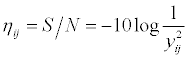(2)

Consequently, the average value of S/N ratio of the ith experiment can be calculated as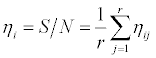(3)

where, i, j represents ith experiment and jth repeated experiment, respectively, i=1 to n, j=1 to r, and n is the total number of experiment, r is the repeated number of each ith experiment, ηij represents each S/N ratio and yij is the experimentally observed value of the ijth experiment. Here, n=16, r=2.

Table 3 shows the experimental measurement results for MRR, The mean value of S/N for each parameter is listed in Table 4. (Ec1 and Ec2 represent empty column 1 and empty column 2, respectively).

No. G Ec1 Ec2 P FS MRR(um/s) S/N(db)
O11 O22 Ave. O11 O22 Ave.
1 320 1 1 20 1.32 3.91 4.15 4.03 11.84 12.36 12.10
2 320 2 2 30 2.08 2.91 3.17 3.04 9.28 10.02 9.65
3 320 3 3 40 2.94 2.87 3.02 2.95 9.16 9.60 9.38
4 320 4 4 50 3.62 3.01 3.19 3.10 9.57 10.08 9.82
5 240 1 2 40 3.62 4.60 4.38 4.49 13.26 12.83 13.04
6 240 2 1 50 2.94 4.76 4.66 4.71 13.55 13.37 13.46
7 240 3 4 20 2.08 4.11 4.47 4.29 12.28 13.01 12.64
8 240 4 3 30 1.32 4.53 4.33 4.43 13.12 12.73 12.93
9 120 1 3 50 2.08 5.04 5.75 5.40 14.05 15.19 14.62
10 120 2 4 40 1.32 5.28 5.25 5.27 14.45 14.40 14.43
11 120 3 1 30 3.62 6.28 6.41 6.35 15.96 16.14 16.05
12 120 4 2 20 2.94 6.00 5.82 5.91 15.56 15.30 15.43
13 80 1 4 30 2.94 4.11 3.16 3.64 12.28 9.99 11.14
14 80 2 3 20 3.62 4.16 3.80 3.98 12.38 11.60 11.99
15 80 3 2 50 1.32 2.52 2.52 2.52 8.03 8.03 8.03
16 80 4 1 40 2.08 2.89 2.86 2.88 9.22 9.13 9.17

Table 3: Experimental layout using L16 orthogonal array with responses (MRR).

Level G Ec1 Ec2 P FS
1 10.239 12.725* 12.696* 13.041* 11.871
2 13.017 12.382 11.538 12.440 11.521
3 15.132* 11.524 12.229 11.505 12.351
4 10.081 11.838 12.007 11.483 12.726*
R 5.051 1.201 1.158 1.558 1.205
Rank 1 4 5 2 3

Table 4: Main effects of S/N.

where, * represents optimal level, and R represents range, its computation formula is as follow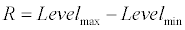(4)

From Table 4, it is observable that abrasive granularity (G), feed pressure (P) and feed speed (FS) are three important factors for USM material removal rate, especially abrasive granularity (G). The further analysis and discussion will be carried out in the following sections.

Discussion and analysis

The main influence of each parameter on MRR is shown in Figure 2. It is observed that the S/N ratio of abrasive granularity (G) has an obvious nonlinear characteristic, and other two S/N ratios (i.e. feed pressure (P) and feed speed (FS) parameters) have almost linear characteristic.

The analysis and discussion for this phenomenon are as follows: for S/N ratio of abrasive granularity, it increased between 80 mesh and 120 mesh, and then decreased between 120 mesh and 320 mesh. It suggests that too small or too big abrasive granularity would not be conducive to the improvement of material removal rate. The reason for this can be explained that too small or too big abrasive granularity would reduce the direct hammering of abrasives by the tool vibration and the cavitation-erosion effect or high velocity impact of free moving abrasive particles on the workpiece surface. For S/N ratio of feed pressure (P), it almost decreased with increasing feed pressure. It can be explained that the vibration frequency and amplitude of tool would be reduced when feed pressure increasing. And for S/N ratio of feed speed (FS), it almost increased with increasing feed speed. It is apparent that increasing feed speed could increase the abrasive mechanical hammering and impact efficiency. The greater value of the mean S/N for each parameter maximizes the MRR value. As seen in Figure 2 and from the data given in Table 4, the MRR reaches the maximum at the 120 mesh for abrasive granularity, 20 N for feed pressure and 3.62 mm/s for feed speed.

Prediction of optimal MRR and confirmation experiment

According to the S/N ratio analysis of section 3.1, the optimal combination was determined for MRR, and the results are presented in Table 4 (marked * in the table). The predictive S/N ratio and optimal mean value for MRR can be calculated as follow [13,18]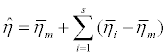(5)

where,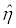represents predictive S/N ratio for optimal combination levels of machining parameters,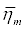represents total mean S/N ratio,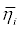represents mean S/N ratio at the optimal levels, and s is the number of significant parameters. Here, s=3.

Using Eq. (5), the predictive S/N ratio for optimal combination levels of machining parameters is calculated as follow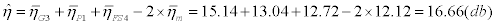Similarly, the predictive optimal mean value of MRR is calculated as follow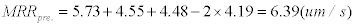After this, a confirmation experiment was performed in order to determine the difference between the predicted and measured values, which is 6.43 um/s. It can be seen that the difference between the predicted and measured values for MRR is negligibly small, with relative error 0.62% only.

ANOVA analysis results

According to data of S/N, the results of ANOVA were given by using SPSS software in Table 5, the significance and importance of the parameters were expressed by the probability values, (i.e. Sig. value) and the percentage contribution values, (i.e. PC value), respectively. When the Sig. value in the ANOVA table is less than 0.05, it is generally considered that the parameters are statistically significant [13,18].

Parameters Process parameters
G P FS Error Total
df 3 3 3 6 15
Seq.SS 70.303 6.940 3.365 6.254 86.862
F 22.484 2.220 1.076
Sig. 0.001 0.187 0.427
PC (%) 80.94 7.98 3.87 7.20

Table 5: Results of ANOVA for S/N.

The Sig. value in Table 5 shows that, except for the abrasive granularity, the other two parameters are statistically insignificant at 5% level, implying that the parameters have insignificant impact on the MRR value. This table also shows that the abrasive granularity (G), feed pressure (P) and feed speed (FS) affect the MRR value by 80.94%, 7.98% and 3.87%, respectively.

#### Optimal Regression Modeling and Verification

Optimal regression modeling

To establish a mathematical relationship between parameters and responses, linear regression analyses were performed in past literature . However, in this study, it is observable that the effect of abrasive granularity on MRR has a nonlinear characteristic and the other two parameters have almost linear characteristic. Consequently, the key step of optimal regression modeling is to find a function to replace the mapping relationship between parameters and response. By using mathematical modeling method, the following equation is selected to fit the measurement data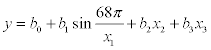(6)

Where, y, x1, x2 and x3 represents MRR, G, P and FS, respectively. And b0, b1, b2, b3 are regression coefficients.

Given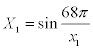, Eq. (6) can be written as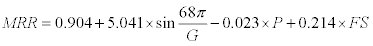(7)

Hence, an optimal regression model was established using regression methods as following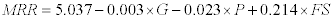(8)

Comparison for two regression models

In order to compare analytical accuracy between optimal regression model and traditional regression model, a traditional regression model was also established using regression methods as following

MRR = 5.037 −0.003×G −0.023×P + 0.214×FS (9)

The significance of two regression models and the each coefficient in the equations were analyzed by ANOVA and tested by the Sig. value. The results of ANOVA of significance test on regression equation are shown in Table 6. It shows that the optimal regression model for MRR is statistically significant at a 95% confidence level, while the traditional regression model for MRR is statistically insignificant at a 95% confidence level.

Parameters Optimal variances sources Traditional variances sources
Regression Error Total Regression Error Total
df 3 12 15 3 12 15
Seq.SS 16.847 2.760 19.608 2.940 16.668 19.608
F 24.413     0.705
Sig. 0.000     0.567

Table 6: ANOVA for two regression equations.

The results of statistics analysis on significance of each coefficient in two equations are shown in Table 7. It indicates that, in the optimal regression model, apart from the coefficients for constant value and feed speed, the coefficients are statistically significant at a 95% confidence level, while in the traditional regression model, apart from the coefficients for constant value, the coefficients are statistically insignificant at a 95% confidence level.

Coefficients T Sig. Coefficients T Sig.
Constant 0.904 1.324 0.210 Constant 5.037 3.566 0.004
sin (68p/G) 5.041 8.132 0.000 G -0.003 -0.969 0.352
P -0.023 -2.170 0.051 P -0.023 -0.883 0.394
FS 0.214 1.550 0.147 FS 0.214 0.631 0.540

Table 7: Regression statistics analysis on significance of each coefficient.

Verification of the regression models

To verify the prediction of the regression models, the sixteen experimental conditions were inserted in two models. The data taken from two regression models were compared with the measurement value, and the results were given as a graph as in Figure 3. As shown in Figure 3, the optimal regression value and measurement value for MRR are very close, while the traditional regression value and measurement value are far apart. The results show that the optimal regression model for MRR responses can be used for determining and estimating the material removal rate in ultrasonic machining process, meanwhile, the fitting accuracy of traditional regression model is very low.

The two regression models were also verified through experiments with new experimental conditions. Test condition and its results were listed in Table 8.

No. G P FS MRR(um/s) (optimal) MRR(um/s) (traditional)
MV RV RE (%) MV RV RE (%)
1 320 20 2.08 3.88 4.01 3.32 3.88 4.06 4.69
2 320 30 2.94 4.18 3.96 5.20 4.18 4.02 3.92
3 240 40 1.32 4.62 4.18 9.47 4.62 3.68 20.36
4 240 50 2.08 4.45 4.12 7.52 4.45 3.61 18.83
5 120 50 2.94 5.25 5.31 1.24 5.25 4.16 20.84
6 120 40 3.62 6.38 5.69 10.81 6.38 4.53 28.97
7 80 30 3.62 3.52 3.28 6.72 3.52 4.88 38.68
8 80 20 1.32 2.86 3.02 5.63 2.86 4.62 61.52

Table 8: Verification results for two regression models.

where, MV, RV and RE represent measurement value, regression value and relative error, respectively.

The results were also presented in Figure 4. As seen in Figure 4 and Table 8, there is a high correlation between the measurement value and the optimal regression value. The actual MRR values are very close to the MRR value calculated from the optimal regression equation. This indicates that the optimal regression model for MRR can predict the process for the actual ultrasonic machining condition.

#### Conclusions

Based on above analyses, the following conclusions were drawn:

1. The effect of abrasive granularity on MRR has an obvious nonlinear characteristic; too small or too big abrasive granularity would not be conducive to the improvement of material removal rate.

2. The effect of abrasive granularity, feed pressure and feed speed on MRR is 80.94%, 7.98% and 3.87%, respectively. Meanwhile, the Sig. value of them is 0.001, 0.187 and 0.428, respectively.

3. The analytical precision of optimal model is quite higher than that of traditional model. It indicates that the optimal regression model can correctly reflect the influence of machining parameters on process response, which can provide a theoretical basis for selection of machining parameters to improve its machining efficiency.

4. The future research directions will be to improve energy efficiency and its machining precision.

#### Acknowledgement

This paper was supported by the research projects: the Research Plan of Nanjing Agricultural University of China (No: 2011G02).

#### References

Select your language of interest to view the total content in your interested language

### Article Usage

• Total views: 14067
• [From(publication date):
December-2015 - Nov 12, 2019]
• Breakdown by view type
• HTML page views : 10000Can't read the image? click here to refresh